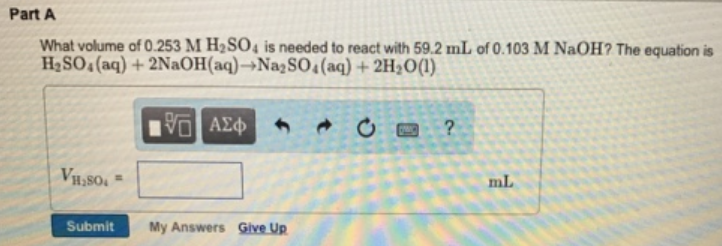# Problem: What volume of 0.253 M H2SO4 is needed to react with 59.2 mL of 0.103 M NaOH? The equation is H2SO4 (aq) + 2NaOH (aq) → Na 2SO4 (aq) + 2H2O (l)

###### FREE Expert Solution
100% (236 ratings)###### Problem Details

What volume of 0.253 M H2SO4 is needed to react with 59.2 mL of 0.103 M NaOH? The equation is

H2SO4 (aq) + 2NaOH (aq) → Na 2SO4 (aq) + 2H2O (l)